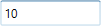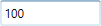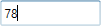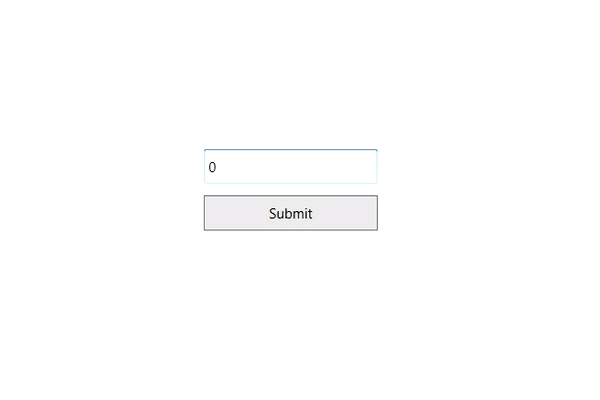# Restriction or Validation in WPF IntegerTextBox

20 May 20215 minutes to read

This section explains how to validate or restrict the IntegerTextBox control value.

## Restrict the value within minimum and maximum value

The Value of the IntegerTextBox can be restricted within the maximum and minimum limits. Once the value has reached the maximum or minimum value , the value does not exceed the limit. We can change the minimum and maximum limits by using the MinValue and MaxValue properties.

You can choose when to validate the maximum and minimum limits while changing the values by using the MinValidation and MaxValidation properties.

• `OnKeyPress` — When MaxValidation or MinValidation properties value is `OnKeyPress`, the value in the IntegerTextBox will be validated shortly after pressing a key. It is not possible to provide any invalid input at all and the value does not exceed the maximum and minimum limits.

• `OnLostFocus` - When MaxValidation or MinValidation properties is `OnLostFocus`, the value in the IntegerTextBox is validated only when the IntegerTextBox loses the focus. After validation, when the value of the IntegerTextBox is greater than the `MaxValue` or less than the `MinValue`, the value will be automatically set to `MaxValue` or `MinValue`.

• MaxValueOnExceedMaxDigit - When you give input greater than specified maximum limit, MaxValueOnExceedMaxDigit property will either retain the old value or reset to maximum limit that is specified. For example, if `MaxValue` is set to 100 and you are trying to input 200. `Value` will changed to 100 when `MaxValueOnExceedMaxDigit` is `true` or 20 will be retained if `MaxValueOnExceedMaxDigit` is `false`.

• MinValueOnExceedMinDigit - When you give input less than specified minimum limit, `MinValueOnExceedMinDigit` property will either it should retain the old value or reset to minimum limit that is specified. For example, if `MinValue` is set to 200 and the `Value` is 205 and you are trying change the value to 20. `Value` will changed to 200 when `MinValueOnExceedMinDigit` is `true` or when `MinValueOnExceedMinDigit` is `false`, Old value 205 will be retained.

NOTE

`MaxValueOnExceedMinDigit` and `MinValueOnExceedMinDigit` properties will be enabled only when the `MaxValidation` and `MinValidation` is set to `OnKeyPress`.

``````<syncfusion:IntegerTextBox x:Name="integerTextBox" Width="150" MaxValue="100" MinValue="10"
MinValueOnExceedMinDigit="True" MaxValueOnExceedMaxDigit="True"
MinValidation="OnKeyPress" MaxValidation="OnLostFocus"/>``````
``````IntegerTextBox integerTextBox = new IntegerTextBox();
integerTextBox.Width = 100;
integerTextBox.Height = 25;
integerTextBox.MinValue = 10;
integerTextBox.MaxValue =100;
integerTextBox.MinValidation = MinValidation.OnKeyPress;
integerTextBox.MaxValidation = MaxValidation.OnLostFocus;
integerTextBox.MinValueOnExceedMinDigit = true;
integerTextBox.MaxValueOnExceedMaxDigit = true;``````

When `MinValidation` value is OnKeyPress, you cannot enter value less than the `MinValue`. If try to enter a value less than the `MinValue`, then the `MinValue` will set to the `Value` property because `MinValueOnExceedMinDigit` is set to `true`.`MaxValidation` is set to OnLostFocus, so the `MaxValidation` will be performed only in the lost focus.The IntegerTextBox doesn’t allow the user input on application runtime when IsReadOnly property is `true`. The user can still select text and display the cursor on the `IntegerTextBox` by setting the IsReadOnlyCaretVisible property to `true`.

``<syncfusion:IntegerTextBox x:Name="integerTextBox" IsReadOnly="True" Value="78" IsReadOnlyCaretVisible="True"/>``
``````IntegerTextBox integerTextBox = new IntegerTextBox();
integerTextBox.Value = 78;## Customize the behavior for invalid value

You can customize how the IntegerTextBox behaves when entered value is not equal to the value of ValidationValue property, using InvalidValueBehavior property. It can be customized by below values,

• `DisplayErrorMessage` - Shows a MessageBox with message” String validation failed” after focus is lost from IntegerTextBox.

• `None` - Validation will not occurs.

• `ResetValue` - Resets the entered value to 0 after focus is lost.

NOTE

By default ValidationValue property value is String.Empty.

``````<syncfusion:DoubleTextBox Width="120" Height="30"
InvalidValueBehavior="DisplayErrorMessage"
ValidationValue="1222"
VerticalAlignment="Center"
HorizontalAlignment="Center" />``````
``````DoubleTextBox doubleTextBox1 = new DoubleTextBox()
{
Height = 30,
Width = 120,
InvalidValueBehavior = InvalidInputBehavior.DisplayErrorMessage,
ValidationValue = "1222",
HorizontalAlignment = HorizontalAlignment.Center,
VerticalAlignment = VerticalAlignment.Center
};``````